# Discussion posts

## MPI Mini fuel calculation

There is a lot that goes into the fuel calculation for the MPI Mini
There are several checks carried out which can have large impacts such as using different AFR targets depending on engine load,
safety checks in case the engine is overheating, alternate maps and multipliers while the engine warms up etc
I will have missed parts of code that affect the fuelling but this is a good start

The main fuel calculation is speed density
Fundamentally this tries to work out how many oxygen molecules are in the combustion chamber, and therefore how many fuel molecules should be added to achieve the desired air to fuel ratio

[I can add a longer SD explanation if people want] . . .

## Intake air temp

Before the main fuel calculation there is a calculation on the IAT (intake air temperature)
This is needed because air (including oxygen) density is directly affected by temperature, not just pressure - cold air is more dense than hot air
Heat soak is caused by the fact that the engine bay and engine are very hot, causing the actual IAT sensor element will be heated up slightly by the radiant heat of the engine which can make it read higher than reality
Inversely, the intake air is heated slightly as it travels through the intake system and into the engine cylinders
How fast the air is moving dictates how much additional heat it will take on, smaller volumes of air moving slowly through the intake (think idle speed) will heat up more than large volumes of air moving very quickly (think full throttle, 6,000 RPM)

This is worked out as follows:
map 71 is estimated IAT given MAP vs RPM, mostly forcing high values at idle: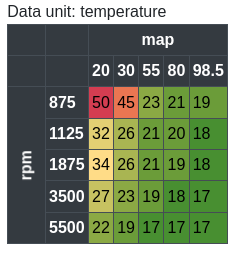map 72 gives a percentage of how much of the real IAT value to use. 100% would use the real IAT sensor value, 0% would use the estimated IAT value, other values scale between the two linearly: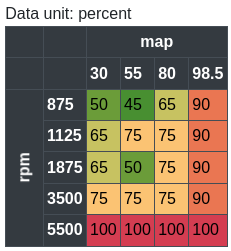In actual ECU code it does the following:

```iat final value =
(
(actual IAT reading - estimated IAT (map 71))
* map 72 percentage value / 100
)
+ estimated IAT (map 71);
```
This basically starts at the estimated IAT value then drags it back towards the real IAT sensor reading by the percentage that we trust the sensor at the moment

## Fuel calculations

Engine size in cc is stored in the ECU calibration e.g. 1275cc
The MAP sensor reading is assumed to be 100kpa if >= 60% throttle is applied, otherwise the actual sensor reading is used

The first main fuel calculation is as follows:
```(engine_size_cc / 4)
* fuel map 73 value
* 8714 (constant)
/
(iat final value * 10000);

To note: the MAP sensor raw reading is 10,000 for 100kpa, the IAT reading is 2932 at 20 degrees C
```
Map 73:The next fuel calc, seems to be working out the fuel mass required to mix with current air mass for 14.5:1 AFR
previous fuel calc * 20 / 290
(same as previous fuel / 14.5 approx)

The next fuel calc, seems like injector flow rate, it is based on map 33, looked up by current car voltage
0x28c = previous fuel calc / injector flow rate
Map 33: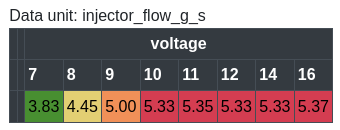Next there is a big recalc involving maps 68, 69, 29, 30, 31 (all are temperature related)
They seem to gather together to make warm up enrichment
Maps 68 and 69 are applied separately/compounding, then maps 29, 30, and 31 are added together and applied as one
The whole of map 68 is effectively multiplied by 1.1 because of map 69

map 68:map 69:new calc = previous fuel calc
* (map_68_value / 100)
* (map_69_looked_up_value_temp_related_dat_414 / 100)

* (map 29 + 30 + 31 + 1000) / 1000
at 10 degrees C -> 300 + 210 + 150 + 1000 = 1660 / 1000 = 1.66
fully warm -> 10 + 80 + 150 + 1000 = 1240 / 1000 = 1.24

fuel_value_dat_3a2 = dat_170 * 100 + 7500
(can't see where dat_170 really gets set, seems related to the map sensor checking, gets set to either 0 or between 75 and 100%)
dat_3a2 gets limited to 10,000 (100%)
* fuel_value_dat_3a2 / 10,000 (as percentage)

* fuel feedback / 10000 (as a percentage)

Next is a pointless operation for this ECU, map 43 has all values set to 100% so nothing will change
* map 43 value / 10000 (as a percentage)

Next the target AFR is used
This can be looked up from map 67 or map 70 depending on TPS/MAP, and is also affected by others things such as if the car overheats past 115 degrees C coolant
* target AFR multiplier / 10000

Map 67: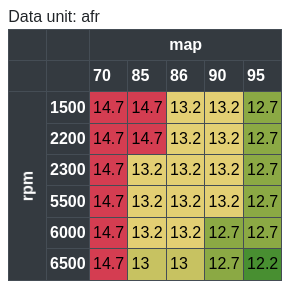Map 70: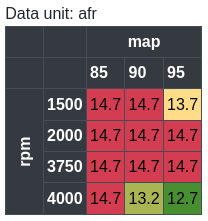There are further steps such as checking immobiliser state, injector times, rev limit etc, but I need to stop somewhere

Contact: email me
Disclaimer: This website is not associated with Rover in any way. This exists because there is no support for these older cars/ECUs